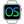HelpWLanguageWLanguage functionsStandard functionsQueue, stack, list and array management functionsArray functionsPrefix syntaxSumPresentationExampleSupported typesSum of durationsSee alsoAddAddLineAddReferenceAddSortedAllFalseAllTrueAnyFalseAnyTrueApplyCopyCountDeleteDeleteAllDeleteDuplicateDeleteLineDistinctFilterInfoInsertInsertLineInsertReferenceIntersectMapMaxMeanMinMoveMoveLineReduceReverseSeekSeekByProcedureShuffleSortSumSwapLineUnionUnionAllDeleteAllFunctions for managing arrays (prefix syntax)
 Supported typesSum of durations WINDEVWEBDEVWINDEV MobileOthers.Sum (Function) In french: .Somme Calculates the sum of the array elements. Example // Sum of elements found in an array of integersMyArray is array of int = [1,2,3]MySum is numericMySum = MyArray.Sum() // MySum = 6 Syntax Calculating the sum of the elements found in a a one-dimensional array (numeric types) = .Sum() : NumericSum of the numeric elements found in the array. : Array variableName of the one-dimensional array of numeric types containing the elements whose sum must be calculated. Calculating the sum of the elements found in a one-dimensional array (structured types) = .Sum() : NumericSum of the values of members. : Array variableName of the one-dimensional array of structured types. The following structured types are allowed: class, dynamic class, structure, dynamic structure.Remark: In an array of dynamic classes or in an array of dynamic structures, the element is ignored if it is not allocated. : Character stringName of the member containing the values that must be used to calculate the sum. Calculating the sum of the elements found in a column of a two-dimensional array = .Sum() : NumericSum of the column values. : Array variableName of the two-dimensional numeric array. : IntegerIndex of the array column containing the values that must be used to calculate the sum.Remarks Supported typesThe following types are supported for the arrays of numeric values and for the members of arrays of structured types: all integer types (on 1, 2, 4 or 8 bytes, signed or unsigned + system integer).real (4 or 8-byte), currency and numeric types.the Duration type.Sum of durationsIf .Sum is used to calculate a sum of durations, all parameters must be of type Duration.Sequence of functionsYou can use array functions in a sequence. The following functions can be used in a sequence: This sequence can be used as a source for a FOR ALL statement or it can end with one of the following functions: Example: gnMean = garrUser.Filter(cbFilter).Map(cbMap).Mean() Business / UI classification: Neutral codeComponent: wd280wm.dll Minimum version required Version 25 This page is also available for…